# Gambler’s Problem

Our agent is playing a game where they can place a bet on whether a coin flip will show heads. If it is heads, then they win the same amount they bet, otherwise, the opposite happens and they lose all they money they bet. The coin lands on heads 40% of the time. The game continues over and over again until the player either has 0 capital (loss) or 100+ capital (win).

This problem can be found in Chapter 4 of Sutton and Barto’s book: Reinforcement Learning: An Introduction.

# Key points of the problem:

• Undiscounted, finite, episodic MDP: Therefore, gamma is 1, and there is a terminal state.
• The state is the gamblers capital.
• Policy is mapping of levels of capital to how much the agent should bet
• Terminal states: 0 capital and 100+ capital.
• Reward of 0 at all states except the 100+ state.

# Solving the problem

In this part of the book, we’re expected to solve this using Value Iteration. In a previous story, I had examined a problem of solving a rental car inventory optimization problem with Policy Iteration.

One issue with Policy Iteration is that it required fully fitting a Value function with a particular policy. As the book suggests, it can be a protracted procedure, whereby the convergence to v_pi occurs only in the limit. I suppose it wouldn’t be as problematic if we didn’t also switch to updating the policy (which then changes the value function) or if we knew that a value function that fully fit a policy resulted in a more efficient update to the policy than if we stopped the iterations earlier.

Instead of looping through a state space with a value function until it converges to v_pi, we can loop just once (updating the values on the way), during which we take the max value from the set of values and assign it to the state being investigated. Thus, every time we sweep through the states, we look at all the places we can go from that position, and simply indicate that the max reward from that position is the maximal value one could expect from that state.Value function for Value Iteration, where the equation is applied iteratively.

One way to think about this formula is that from a given state, there exists many actions. So we accumulate the values for how much each action would get in terms of expected value, and simply assign the value of the state to that of the action which returned the highest value. This formula is similar to the Policy Evaluation update except for this important fact of taking the maximum over all actions.

Another way to think about is that we’ve taken the Bellman Optimality equation and turned it into an update rule.

Unlike Policy Iteration, we don’t use or build an explicit policy at each step, exclusively working within the value space. We go from value function, to value function, to value function. At the end of the process, we are guaranteed to have the value function for the optimal policy. It’s akin to a Modified Policy Iteration with k = 1, since we are taking the max over the next step.

One thing important to note is that the interim value functions between the initial value function and the optimal value function may not correspond to any policy. This is different than Policy Iteration where at every step value function is calculated for a particular policy.The value functions continue to improve till their reach the optimal value function, but the interim values may not correspond to any particular real policy.

In essence, it combines one step of policy evaluation with policy improvement.

There’s two points in this problem where I got stuck.

## Value Function

When calculating the value function, I believed I was supposed to mimic the Bellman Equation exaction.

`def expectedReturn(state, action, stateValue):    outcomes = [0, 1]    returns = 0    reward = 0    for index in outcomes:        if (index == 0):            nextState = state - action            probs = 1 - PROB_HEADS            reward = 0        else:            nextState = state + action            probs = PROB_HEADS            if (index > 100):                 reward = 1        returns += reward + (probs * stateValue[nextState])    return returns`

However when I was comparing my diagram to the one in the book, I noticed very different scales. Their value estimates were all below one, while mine was above. It’s not 100% evident to my why we’re supposed to not include the reward in the calculation. My hunch is the following:

• Calculating rewards on the way is less useful here, as there are no rewards at all until you reach the final state? In other problems, I’ve noticed that while a reward might be at the end, typically there are at least negative penalties on the way (i.e. to penalize taking a long time). I’m not entirely convinced by this argument.
• Is the value estimates the probability of winning the game?

My approach to calculating the return was doing a more elaborate separate function in order to allow for some flexibility for adding functionality in the future. The idea was to iterate through the two outcomes of flipping the count and iteratively add their values.

`def expectedReturn(state, action, stateValue):    outcomes = [0, 1]    returns = 0    for index in outcomes:        if (index == 0):            nextState = state - action            probs = 1 - PROB_HEADS        else:            nextState = state + action            probs = PROB_HEADS        returns += probs * stateValue[nextState]    return returns`

However, this is rather long, unnecessarily elaborate and could easily be shaved down by doing the calculations inline, as was done in the actual solution:

`for action in actions:        expectedReturns.append((1 - PROB_HEADS) * stateValues[state - action] + PROB_HEADS * stateValues[state + action])`

I decided to make a simple function since I use the same line again.

`def expReturn(state, action, stateValue):    return (1 - PROB_HEADS) * (stateValue[state - action]) + PROB_HEADS * (stateValue[state + action])`

Ultimately, the final state value function did end up the same as the one from the book.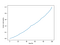Value function for p=0.4 and s ϵ S where S is all integers from 0 to 100

## Optimal Policy

What’s curious about the outcome is that even though it mimics the solution, it still feels a bit unsatisfying. Why does the policy choose zero for certain situations? The value function looks exactly the same as the solution, yet the policy looks very different from the book.

In the solution code, Shangton writes the solution can’t be replicated because of ties. I should’ve recognized that hint earlier as I got stuck trying to search online as to why there might be an issue.

A tutorial from 2006 suggests that the actual graph to the policy might look a little different and that the sort of big spikes/troughs seen in the graph could be caused by noise and a result of roundup errors of the floating point limitations of CPUs. They also showed that their graph ended up looking quite a bit different than the one from the Sutton and Barto Reinforcement Learning book.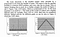From A Short Tutorial on Reinforcement Learning by Chengcheng Li and Larry Pyeatt (2006)

Upon further inspection of the expected returns for each action from a given state, I realized that some of the values had duplicates. For example State 4 has five actions it could choose from with five different values. Betting nothing and betting four have the same results.

`state 4 - action 0: 0.0130229033514state 4 - action 1: 0.0127258811206state 4 - action 2: 0.0125767600852state 4 - action 3: 0.0124992467234state 4 - action 4: 0.0130229033514`

Thus, when I attempt to naively return the max argument, numpy will encounter a tie at 0 and the last position, selecting the first element encountered as the tie breaker.

If I opt to instead select the highest index value and highest number, the graph will change to something like a triangle. I should note that I got stuck on this particular point because my conditional was greater than instead of greater than equals to, which effectively meant that if two values had the same maximal value and one of them was the zero index, the zero index would always win out because the policy was initialized with all zeros.

`policy = np.zeros(GOAL + 1)for s in states[1:GOAL]:    expReturns = []    actions = np.arange(min(state, GOAL - s) + 1)    for a in actions:        expReturns.append(expectedReturn(s, a, stateValues))    for i, value in enumerate(expectedReturns):        if (value >= expReturns[int(policy[s])]):            policy[state] = index`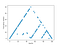s ϵ S where S is all integers from 0 to 100

The policy become more uniform if I select 99 as the goal of the program and use the higher index as tie-breaker for maxing over argument index values.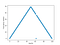s ϵ S where S is all integers from 0 to 99

It’s still not 100% clear to me why they have such different results as I would’ve expected the policy to act pretty consistently independent of state size.

One of the keys might be at the end of the problem, where they comment on the graphical diagram of the final policy:

This policy is optimal, but not unique. In fact, there is a whole family of optimal policies, all corresponding to ties for the argmax action selection with respect to the optimal value function.

So, perhaps it is a case that some of the previous diagrams where the optimal action is to do nothing (0) were acceptable solutions.

Ultimately it seemed liked the best overall solution was to either make a maximal bet with what you had in your capital or to not bet at all.

# Other probabilities

From the Li/Pyeatt tutorial, it was mentioned that the policy from between 0 to 0.5 would appear triangle like given a particular number of states, specifically:

The local maximum is the smallest integer value divisible by a polynomial of two from the number of states.The reason is that the gambler problem is a discrete MDP problem, and every state has an integer value as capital.

We can test out their explanation by attempting a version with 128 states, and 127. Lo and behold, we end up with a policies that look wildly different.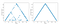Left: 128 states, a polynomial of 2 whereas on the Right: 127 states

I was curious to see what happens if I adjust the probability and keep to the same behavior of selecting the maximal index value to resolve ties.

When the probability of rolling heads is 0.75, the agent will prefer to make small bets of 1 until it has about around 40 capital or so, at which point it will progressively increase it’s bet. At around 70 capital and beyond, it will bet as much as it can to finish the game.